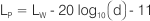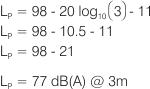Technical LibraryNoise & Acoustic

dB(A) Information

Noise Levels - Calculation and use of the dB(A)

The human ear is more sensitive to some frequencies than others. In particular low frequency noises sound quieter than high frequency noises. In the 1930’s, experiments were carried out on 11 people by Harvey Fletcher at the Bell Telephone Laboratories in New York to determine how loud tones of different frequencies sounded subjectively.

The ‘A’ frequency weighting or more commonly dB(A) is based on how loud various tones played at different frequencies sound compared to a tone of 40 dB, at 1000 Hz. The ‘A’ frequency weighting corrections are shown below.

Octave Band Centre Frequency, Hz 63 125 250 500 1k 2k 4k 8k
‘A’ frequency weighting corrections -26 -16 -9 -3 0 1 1 -1

The ‘A’ frequency weighting suggests that if a tone of 40 dB is played at 1000 Hz, a 40 dB tone played at 63 Hz would sound 26 dB quieter, or be 14 dB(A). Due to its simplicity and convenience, the ‘A’ frequency weighting has become popular and is now used for many different noise sources at different levels.

In fact, most legislation regarding noise is written using dB(A)s, in addition nearly all manufacturers of fans and other noise generating machines quote their noise levels in dB(A)s at 1, 1.5, or 3 metres assuming spherical distribution. It is therefore important that we understand the ‘A’ frequency weighting and how dB(A)s are calculated.

### Information on Fan Noise Test Standards

Where noted in the product data pages within this catalogue fan noise levels are tested to BS848 Part 2: 1985 “Fans for general purposes. Methods of noise testing”.

This test standard describes methods that may be applied to calculate the sound power level of fans. That is, the In-Duct method, the Reverberant Room method and the Free Field method. The sound pressure level of a product is measured using one of these test methods. A calculation is then used to convert the measured sound pressure levels to sound power levels.

### A Cautious Word on the use of dB(A) Levels

The dB(A) sound pressure level is used almost universally to describe the noise level of many items of noise emitting machinery. However, published dB(A) sound pressure levels should be used for comparative purposes only, they are not designed to reflect actual installed noise levels. The assumptions that are used to calculate the dB(A) are rarely replicated in real life situations and, therefore, published dB(A) values will not necessarily represent the actual noise levels that may be experienced on site.

In order to determine the actual dB(A) sound pressure level that may be expected from an installation, an acoustic analysis of the system, using sound power levels and taking into account the surrounding acoustic environment, should be performed.

### Calculating dB(A) Noise Levels

Published dB(A), or ‘A’ frequency weighted, sound pressure levels are theoretical values. These are, in fact, calculated from the sound power level data and are quoted at a specified distance i.e. 1, 1.5, or 3 metres. For example, using the Fantech model AP0804AP10/23 (duty 7000 l/s @ 80 Pa, inlet side), by applying an ‘A’ frequency weighting correction to the fan sound power levels for each frequency and then logarithmically adding the values from left to right the resultant overall sound power level for this unit will be 98 dB(A). A further calculation is required to convert this value from the ‘A’ weighted sound power level to an ‘A’ weighted sound pressure level at a prescribed distance from the noise source i.e. 77 dB(A) @ 3m. See page N-14 for a detailed example of this calculation.

### 1. ‘A’ weighting corrections

In-duct Sound Power levels, LwdB re 1pW
Frequency (Hz) 63 125 250 500 1k 2k 4k 8k
AP0804AP10/23 Inlet 94 88 95 94 94 91 88 80
'A' frequency weighting correction -26 -16 -9 -3 0 1 1 -1
dB(A) Sound Power level 68 72 86 91 94 92 89 79

### 2. Addition of sound levels

(Refer also to Page H-33, Figure 17 in the Fans by Fantech catalogue).

68 + 72   4   1.5   73.5
73.5 + 86   12.5   0.2   86.2
86.2 + 91   4.8   1.2   92.2
92.2 + 94   1.8   2.2   96.2
96.2 + 92   4.2   1.4   97.6
97.6 + 89   8.6   0.6   98.2
98.2 + 79   19.2   0   98.2

98.2 dB(A) is rounded to 98 dB(A).

### 3. Converting Sound Power to Sound Pressure

To convert this 'A' weighted sound "power" level to an 'A' weighted sound "pressure" level (which is calculated for a specified distance from the source) the following equation is used:Where:

• Lw = Sound Power Level re 10-12W (dB)
• Lp = Sound Pressure Level re 20mPa (dB)
• d = Distance from fan in metres (m)
Therefore, to determine the dB(A) sound pressure level at a distance of 3m:Note that the above calculation assumes that the fan behaves as a point source of noise, that the noise radiates in all directions equally, and that no reflected sound is present.